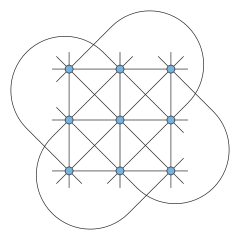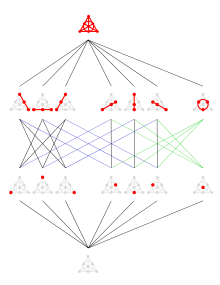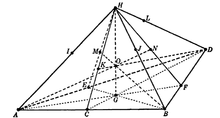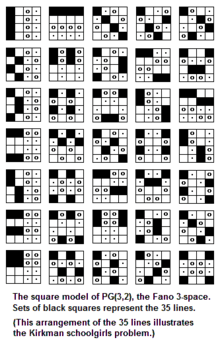# Finite geometryFinite affine plane of order 2, containing 4 points and 6 lines. Lines of the same color are "parallel".

A finite geometry is any geometric system that has only a finite number of points. The familiar Euclidean geometry is not finite, because a Euclidean line contains infinitely many points. A geometry based on the graphics displayed on a computer screen, where the pixels are considered to be the points, would be a finite geometry. While there are many systems that could be called finite geometries, attention is mostly paid to the finite projective and affine spaces because of their regularity and simplicity. Other significant types of finite geometry are finite Möbius or inversive planes and Laguerre planes, which are examples of a general type called Benz planes, and their higher-dimensional analogs such as higher finite inversive geometries.

Finite geometries may be constructed via linear algebra, starting from vector spaces over a finite field; the affine and projective planes so constructed are called Galois geometries. Finite geometries can also be defined purely axiomatically. Most common finite geometries are Galois geometries, since any finite projective space of dimension three or greater is isomorphic to a projective space over a finite field (that is, the projectivization of a vector space over a finite field). However, dimension two has affine and projective planes that are not isomorphic to Galois geometries, namely the non-Desarguesian planes. Similar results hold for other kinds of finite geometries.

## Finite affine and projective planesFinite affine plane of order 3, containing 9 points and 12 lines.

The following remarks apply only to finite planes. There are two main kinds of finite plane geometry: affine and projective. In an affine plane, the normal sense of parallel lines applies. In a projective plane, by contrast, any two lines intersect at a unique point, so parallel lines do not exist. Both finite affine plane geometry and finite projective plane geometry may be described by fairly simple axioms.

An affine plane geometry is a nonempty set X (whose elements are called "points"), along with a nonempty collection L of subsets of X (whose elements are called "lines"), such that:

1. For every two distinct points, there is exactly one line that contains both points.
2. Playfair's axiom: Given a lineand a pointnot on, there exists exactly one linecontainingsuch that3. There exists a set of four points, no three of which belong to the same line.

The last axiom ensures that the geometry is not trivial (either empty or too simple to be of interest, such as a single line with an arbitrary number of points on it), while the first two specify the nature of the geometry.

The simplest affine plane contains only four points; it is called the affine plane of order 2. (The order of an affine plane is the number of points on any line, see below.) Since no three are collinear, any pair of points determines a unique line, and so this plane contains six lines. It corresponds to a tetrahedron where non-intersecting edges are considered "parallel", or a square where not only opposite sides, but also diagonals are considered "parallel". More generally, a finite affine plane of order n has n2 points and n2 + n lines; each line contains n points, and each point is on n + 1 lines. The affine plane of order 3 is known as the Hesse configuration.

A projective plane geometry is a nonempty set X (whose elements are called "points"), along with a nonempty collection L of subsets of X (whose elements are called "lines"), such that:

1. For every two distinct points, there is exactly one line that contains both points.
2. The intersection of any two distinct lines contains exactly one point.
3. There exists a set of four points, no three of which belong to the same line.Duality in the Fano plane: Each point corresponds to a line and vice versa.

An examination of the first two axioms shows that they are nearly identical, except that the roles of points and lines have been interchanged. This suggests the principle of duality for projective plane geometries, meaning that any true statement valid in all these geometries remains true if we exchange points for lines and lines for points. The smallest geometry satisfying all three axioms contains seven points. In this simplest of the projective planes, there are also seven lines; each point is on three lines, and each line contains three points.This particular projective plane is sometimes called the Fano plane. If any of the lines is removed from the plane, along with the points on that line, the resulting geometry is the affine plane of order 2. The Fano plane is called the projective plane of order 2 because it is unique (up to isomorphism). In general, the projective plane of order n has n2 + n + 1 points and the same number of lines; each line contains n + 1 points, and each point is on n + 1 lines.

A permutation of the Fano plane's seven points that carries collinear points (points on the same line) to collinear points is called a collineation of the plane. The full collineation group is of order 168 and is isomorphic to the group PSL(2,7) ≈ PSL(3,2), which in this special case is also isomorphic to the general linear group GL(3,2) ≈ PGL(3,2).

### Order of planes

A finite plane of order n is one such that each line has n points (for an affine plane), or such that each line has n + 1 points (for a projective plane). One major open question in finite geometry is:

Is the order of a finite plane always a prime power?

This is conjectured to be true.

Affine and projective planes of order n exist whenever n is a prime power (a prime number raised to a positive integer exponent), by using affine and projective planes over the finite field with n = pk elements. Planes not derived from finite fields also exist, but all known examples have order a prime power.

The best general result to date is the Bruck–Ryser theorem of 1949, which states:

If n is a positive integer of the form 4k + 1 or 4k + 2 and n is not equal to the sum of two integer squares, then n does not occur as the order of a finite plane.

The smallest integer that is not a prime power and not covered by the Bruck–Ryser theorem is 10; 10 is of the form 4k + 2, but it is equal to the sum of squares 12 + 32. The non-existence of a finite plane of order 10 was proven in a computer-assisted proof that finished in 1989 – see (Lam 1991) for details.

The next smallest number to consider is 12, for which neither a positive nor a negative result has been proved.

### History

Individual examples can be found in the work of Thomas Penyngton Kirkman (1847) and the systematic development of finite projective geometry given by von Staudt (1856).

The first axiomatic treatment of finite projective geometry was developed by the Italian mathematician Gino Fano. In his work on proving the independence of the set of axioms for projective n-space that he developed, he considered a finite three dimensional space with 15 points, 35 lines and 15 planes (see diagram), in which each line had only three points on it.

In 1906 Oswald Veblen and W. H. Bussey described projective geometry using homogeneous coordinates with entries from the Galois field GF(q). When n + 1 coordinates are used, the n-dimensional finite geometry is denoted PG(n, q). It arises in synthetic geometry and has an associated transformation group.

## Finite spaces of 3 or more dimensions

For some important differences between finite plane geometry and the geometry of higher-dimensional finite spaces, see axiomatic projective space. For a discussion of higher-dimensional finite spaces in general, see, for instance, the works of J.W.P. Hirschfeld. The study of these higher-dimensional spaces (n ≥ 3) has many important applications in advanced mathematical theories.

### Axiomatic definition

A projective space S can be defined axiomatically as a set P (the set of points), together with a set L of subsets of P (the set of lines), satisfying these axioms :

• Each two distinct points p and q are in exactly one line.
• Veblen's axiom: If a, b, c, d are distinct points and the lines through ab and cd meet, then so do the lines through ac and bd.
• Any line has at least 3 points on it.

The last axiom eliminates reducible cases that can be written as a disjoint union of projective spaces together with 2-point lines joining any two points in distinct projective spaces. More abstractly, it can be defined as an incidence structure (P, L, I) consisting of a set P of points, a set L of lines, and an incidence relation I stating which points lie on which lines.

Obtaining a finite projective space requires one more axiom:

• The set of points P is a finite set.

In any finite projective space, each line contains the same number of points and the order of the space is defined as one less than this common number.

A subspace of the projective space is a subset X, such that any line containing two points of X is a subset of X (that is, completely contained in X). The full space and the empty space are always subspaces.

The geometric dimension of the space is said to be n if that is the largest number for which there is a strictly ascending chain of subspaces of this form:### Algebraic construction

A standard algebraic construction of systems satisfies these axioms. For a division ring D construct an (n + 1)-dimensional vector space over D (vector space dimension is the number of elements in a basis). Let P be the 1-dimensional (single generator) subspaces and L the 2-dimensional (two independent generators) subspaces (closed under vector addition) of this vector space. Incidence is containment. If D is finite then it must be a finite field GF(q), since by Wedderburn's little theorem all finite division rings are fields. In this case, this construction produces a finite projective space. Furthermore, if the geometric dimension of a projective space is at least three then there is a division ring from which the space can be constructed in this manner. Consequently, all finite projective spaces of geometric dimension at least three are defined over finite fields. A finite projective space defined over such a finite field has q + 1 points on a line, so the two concepts of order coincide. Such a finite projective space is denoted by PG(n, q), where PG stands for projective geometry, n is the geometric dimension of the geometry and q is the size (order) of the finite field used to construct the geometry.

In general, the number k-dimensional subspaces of PG(n, q) is given by the product:which is a Gaussian binomial coefficient, a q analogue of a binomial coefficient.

### Classification of finite projective spaces by geometric dimension

• Dimension 0 (no lines): The space is a single point and is so degenerate that it is usually ignored.
• Dimension 1 (exactly one line): All points lie on the unique line, called a projective line.
• Dimension 2: There are at least 2 lines, and any two lines meet. A projective space for n = 2 is a projective plane. These are much harder to classify, as not all of them are isomorphic with a PG(d, q). The Desarguesian planes (those that are isomorphic with a PG(2, q)) satisfy Desargues's theorem and are projective planes over finite fields, but there are many non-Desarguesian planes.
• Dimension at least 3: Two non-intersecting lines exist. The Veblen–Young theorem states in the finite case that every projective space of geometric dimension n ≥ 3 is isomorphic with a PG(n, q), the n-dimensional projective space over some finite field GF(q).

### The smallest projective three-spacePG(3,2) but not all the lines are drawn

The smallest 3-dimensional projective space is over the field GF(2) and is denoted by PG(3,2). It has 15 points, 35 lines, and 15 planes. Each plane contains 7 points and 7 lines. Each line contains 3 points. As geometries, these planes are isomorphic to the Fano plane.Square model of Fano 3-space

Every point is contained in 7 lines. Every pair of distinct points are contained in exactly one line and every pair of distinct planes intersects in exactly one line.

In 1892, Gino Fano was the first to consider such a finite geometry.

#### Kirkman's Schoolgirl Problem

PG(3,2) can arise as a solution of Kirkman's schoolgirl problem, which states: “Fifteen schoolgirls walk each day in five groups of three. Arrange the girls’ walk for a week so that in that time, each pair of girls walks together in a group just once.” (See answer in external link.) There are 35 different combinations for the girls to walk together. There are also 7 days of the week, and 3 girls in each group. Two of the seven non-isomorphic solutions to this problem can provide a visual representation of the Fano 3-space. Some diagrams for this problem can be found at :

Each color represents the day of the week (seven colors, blue, green, yellow, purple, red, black, and orange). The definition of a Fano space says that each line is on three points. The figure represents this showing that there are 3 points for every line. This is the basis for the answer to the schoolgirl problem. This figure is then rotated 7 times. There are 5 different lines for each day, multiplied by 7 (days) and the result is 35. Then, there are 15 points, and there are also 7 starting lines on each point. This then gives a representation of the Fano 3-space, PG(3,2).